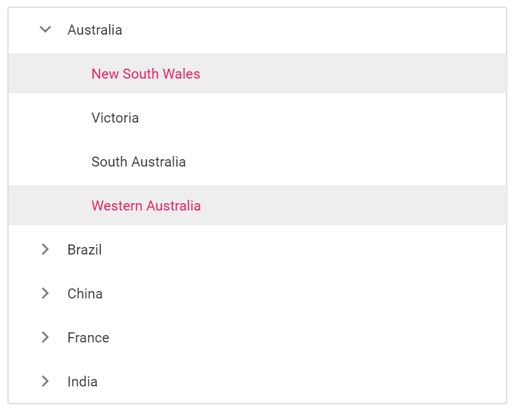# Multi Selection

25 Feb 202224 minutes to read

Selection provides an interactive support and highlights the node that you select. Selection can be done through simple mouse down or keyboard interaction.

The TreeView also supports selection of multiple nodes by setting allowMultiSelection to true.

To multi-select, press and hold CTRL key and click the desired nodes. To select range of nodes, press and hold the SHIFT key and click the nodes.
In the following example, the `allowMultiSelection` property is enabled.

Multi selection is not applicable through touch interactions.

``````<ejs-treeview id="treedata" allowMultiSelection="true">
<e-treeview-fields dataSource="ViewBag.dataSource" id="id" parentId="pid" text="name" hasChildren="hasChild" expanded="expanded" selected="is_selected"></e-treeview-fields>
</ejs-treeview>``````
``````using System;
using System.Collections.Generic;
using System.Linq;
using Microsoft.AspNetCore.Mvc;

namespace EJ2CoreSampleBrowser.Controllers.TreeView
{
public partial class TreeViewController : Controller
{
public IActionResult MultiSelection()
{
List<object> treedata = new List<object>();
{
id = 1,
name = "Australia",
hasChild = true,
expanded = true
});
{
id = 2,
pid = 1,
name = "New South Wales",
is_selected=true

});
{
id = 3,
pid = 1,
name = "Victoria"
});

{
id = 4,
pid = 1,
name = "South Australia"
});
{
id = 6,
pid = 1,
name = "Western Australia",
is_selected = true

});
{
id = 7,
name = "Brazil",
hasChild = true
});
{
id = 8,
pid = 7,
name = "Paraná"
});
{
id = 9,
pid = 7,
name = "Ceará"
});
{
id = 10,
pid = 7,
name = "Acre"
});
{
id = 11,
name = "China",
hasChild = true
});
{
id = 12,
pid = 11,
name = "Guangzhou",
});
{
id = 13,
pid = 11,
name = "Shanghai"
});
{
id = 14,
pid = 11,
name = "Beijing"
});
{
id = 15,
pid = 11,
name = "Shantou"

});
{
id = 16,
name = "France",
hasChild = true

});
{
id = 17,
pid = 16,
name = "Pays de la Loire"

});
{
id = 18,
pid = 16,
name = "Aquitaine"

});
{
id = 19,
pid = 16,
name = "Brittany"

});
{
id = 20,
pid = 16,
name = "Lorraine"
});
{
id = 21,
name = "India",
hasChild = true

});
{
id = 22,
pid = 21,
name = "Assam"

});
{
id = 23,
pid = 21,
name = "Bihar"
});
{
id = 24,
pid = 21,

});
ViewBag.dataSource = treedata;
return View();
}
}
}``````

Output be like the below.## Selected nodes

You can get or set the selected nodes in TreeView at initial rendering and dynamically by using the selectedNodes property. It will return the selected node’s ID as an array.

In the following example, New South Wales and Western Australia nodes are selected at initial rendering. When a node is selected, the selected node’s ID is displayed in alert.

``````<ejs-treeview id="treedata" allowMultiSelection="true" selectedNodes="ViewBag.selectedNodes" nodeSelected="nodeSelected">
<e-treeview-fields dataSource="ViewBag.dataSource" id="id" parentId="pid" text="name" hasChildren="hasChild" expanded="expanded" selected="is_selected"></e-treeview-fields>
</ejs-treeview>

<script>
function nodeSelected(args) {
alert("The selected node's id: " + this.selectedNodes); // To alert the selected node's id.
}
</script>``````
``````using System;
using System.Collections.Generic;
using System.Linq;
using Microsoft.AspNetCore.Mvc;

namespace EJ2CoreSampleBrowser.Controllers.TreeView
{
public partial class TreeViewController : Controller
{
public IActionResult MultiSelection()
{
List<object> treedata = new List<object>();
{
id = 1,
name = "Australia",
hasChild = true,
expanded = true
});
{
id = 2,
pid = 1,
name = "New South Wales"
});
{
id = 3,
pid = 1,
name = "Victoria"
});

{
id = 4,
pid = 1,
name = "South Australia"
});
{
id = 6,
pid = 1,
name = "Western Australia",
is_selected = true

});
{
id = 7,
name = "Brazil",
hasChild = true
});
{
id = 8,
pid = 7,
name = "Paraná"
});
{
id = 9,
pid = 7,
name = "Ceará"
});
{
id = 10,
pid = 7,
name = "Acre"
});
{
id = 11,
name = "China",
hasChild = true
});
{
id = 12,
pid = 11,
name = "Guangzhou",
});
{
id = 13,
pid = 11,
name = "Shanghai"
});
{
id = 14,
pid = 11,
name = "Beijing"
});
{
id = 15,
pid = 11,
name = "Shantou"

});
{
id = 16,
name = "France",
hasChild = true

});
{
id = 17,
pid = 16,
name = "Pays de la Loire"

});
{
id = 18,
pid = 16,
name = "Aquitaine"

});
{
id = 19,
pid = 16,
name = "Brittany"

});
{
id = 20,
pid = 16,
name = "Lorraine"
});
{
id = 21,
name = "India",
hasChild = true

});
{
id = 22,
pid = 21,
name = "Assam"

});
{
id = 23,
pid = 21,
name = "Bihar"
});